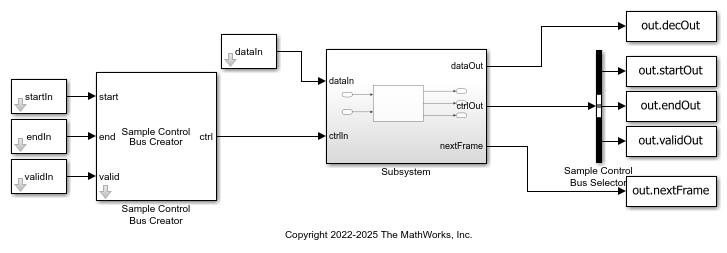# Decode and Recover Message Using CCSDS LDPC Decoder

This example shows how to decode and recover a message from a codeword using an LDPC decoder designed according to the Consultative Committee for Space Data Systems (CCSDS) standard. This example comprises two Simulink® models, each containing a CCSDS LDPC Decoder block. Use the `ccsdsLDPCModelBase` model for the `(8160,7136) LDPC` configuration and the `ccsdsLDPCModelAR4JA` model for the `AR4JA LDPC` configuration. To verify the behavior of the block, compare the output of the model with the input provided to the encoder function. The CCSDS LDPC Decoder block used in these models supports HDL code generation.

### Set Up Input Variables

Set up workspace variables to generate inputs. These values are tunable and you can modify them according to your requirement. The block length index must be in the range [0, 2] and it is applicable only when `configType` is set to `'AR4JA LDPC'`. The code rate index must be in the range [0, 2] and it is applicable only when `configType` is set to `'AR4JA LDPC'`.

```numFrames = 2; configType = 'AR4JA LDPC'; % Select the configuration type as % '(8160,7136) LDPC' or 'AR4JA LDPC' blkLenIdx = [0 2]; codeRateIdx = [1 0]; nIter = 8; % Number of iterations in the range [1, 63] EbNo = 4; ```

### Generate Input Data

Generate input data for the Simulink® model and the MATLAB functions that you use in this example.

```blkLenSet = [1024 4096 16384]; codeRateSet = {'1/2' '2/3' '4/5'}; msg = {numFrames}; decSampleIn = []; decValidIn = []; decStartIn = []; decEndIn =[]; blockLenIn = []; codeRateIn = []; for ii = 1:numFrames % Input and output codeword length calculation if strcmpi(configType,'AR4JA LDPC') blockLen = blkLenSet(blkLenIdx(ii)+1); codeRate = codeRateSet{codeRateIdx(ii)+1}; R = str2num(codeRate); %#ok<*ST2NM> else blockLen = 7136; R = 7/8; end bps = 2; % Input bits generation msg{ii} = (randi([0 1],blockLen,1)); % Data before encoding % LDPC encoding G = generatorMatrix(configType,blockLen,R); encData = satcom.internal.ccsds.tmldpcEncode(msg{ii},G); % Encoded data % Symbol mapping symOut = pskmod(double(encData),bps); % Channel addition - AWGN channel EsNo = EbNo + 10*log10(bps); snrdB = EsNo + 10*log10(R); % Noise in dB noiseVar = 1./(10.^(snrdB/10)); chan = comm.AWGNChannel('NoiseMethod','Variance','Variance',noiseVar); rxData = chan(symOut); % Symbol demapping demodOut = pskdemod(rxData,bps,'OutputType','llr', ... 'NoiseVariance',noiseVar); ldpcLen = length(demodOut); decFrameGap = nIter*5000; % Maximum frame gap considering all block lengths and code rates decSampleIn = fi([decSampleIn demodOut.' zeros(1,decFrameGap)],1,4,0); %#ok<*AGROW> decStartIn = logical([decStartIn 1 zeros(1,ldpcLen-1) zeros(1,decFrameGap)]); decEndIn = logical([decEndIn zeros(1,ldpcLen-1) 1 zeros(1,decFrameGap)]); decValidIn = logical([decValidIn ones(1,ldpcLen) zeros(1,decFrameGap)]); blockLenIn = fi([blockLenIn repmat(blkLenIdx(ii),1,ldpcLen) zeros(1,decFrameGap)],0,2,0); codeRateIn = fi([codeRateIn repmat(codeRateIdx(ii),1,ldpcLen) zeros(1,decFrameGap)],0,2,0); end dataIn = decSampleIn.'; validIn = decValidIn; startIn = decStartIn; endIn = decEndIn; blkLenIdxIn = blockLenIn; codeRateIdxIn = codeRateIn; simTime = length(decValidIn) + decFrameGap; if strcmpi(configType,'AR4JA LDPC') modelName = 'ccsdsLDPCModelAR4JA.slx'; else modelName = 'ccsdsLDPCModelBase.slx'; end ```

Running the model imports the input signal variables `dataIn`, `startIn`, `endIn`, `validIn`, `blkLenIdxIn`, `codeRateIdxIn`, and `simTime` from the script. The model exports a stream of decoded output samples `dataOut` and a control bus containing `startOut`, `endOut`, and `validOut` signals to the MATLAB workspace.

```open_system(modelName); out = sim(modelName); ```### Compare Simulink Model Output with MATLAB Function Input

Compare the output of the model with the encoder function input data.

```startIdx = find(squeeze(out.startOut)); endIdx = find(squeeze(out.endOut)); validOut = (squeeze(out.validOut)); decData = squeeze(out.decOut); fprintf('Decoded data with the following configuration: \n'); for ii = 1:numFrames idx = startIdx(ii):endIdx(ii); decHDL = decData(idx); validHDL = validOut(idx); HDLOutput = logical(decHDL(validHDL)); error = sum(abs(logical(msg{ii})-HDLOutput(:))); fprintf('Frame: %d, The Simulink model output and the MATLAB function input differs by %d bits\n', ii, error); end close_system(modelName,0); ```
```Decoded data with the following configuration: Frame: 1, The Simulink model output and the MATLAB function input differs by 0 bits Frame: 2, The Simulink model output and the MATLAB function input differs by 0 bits ```